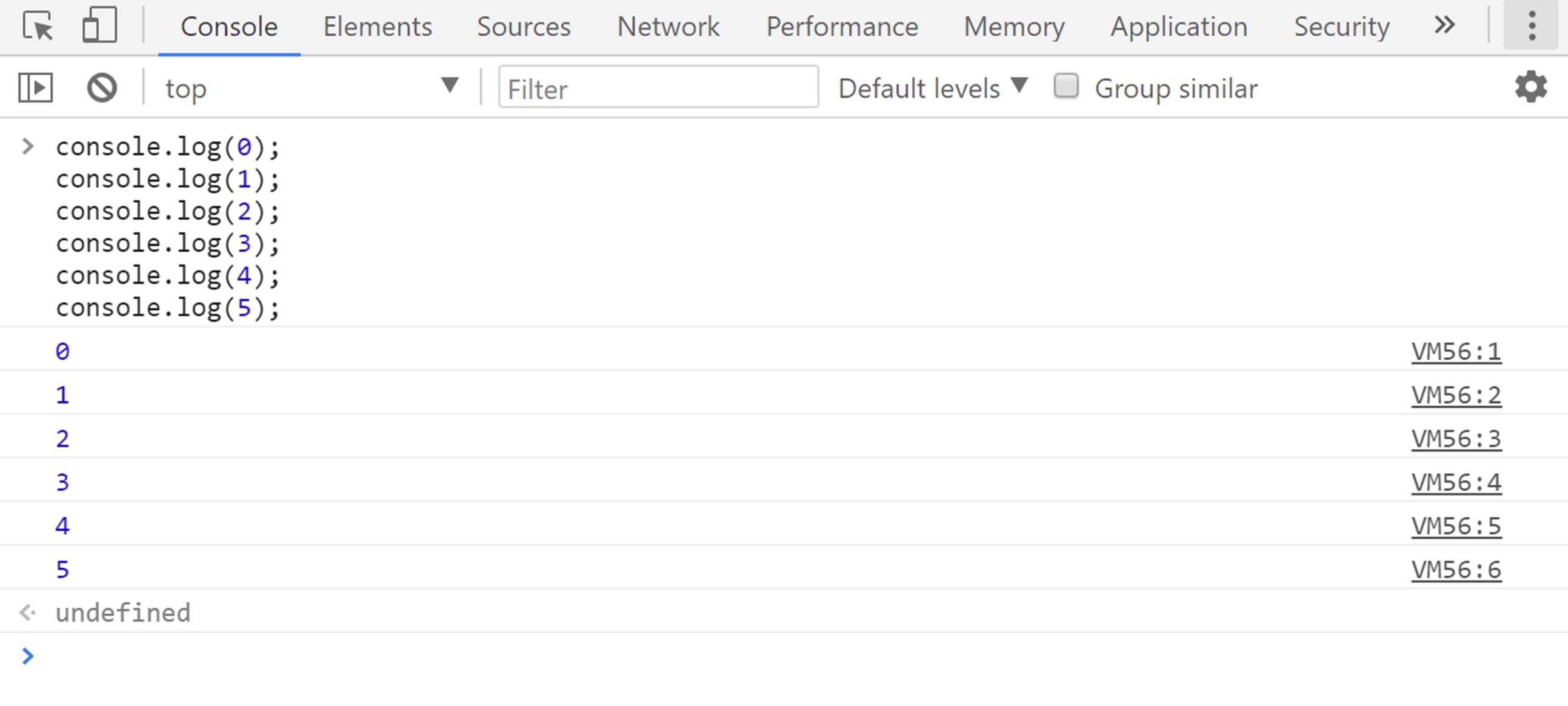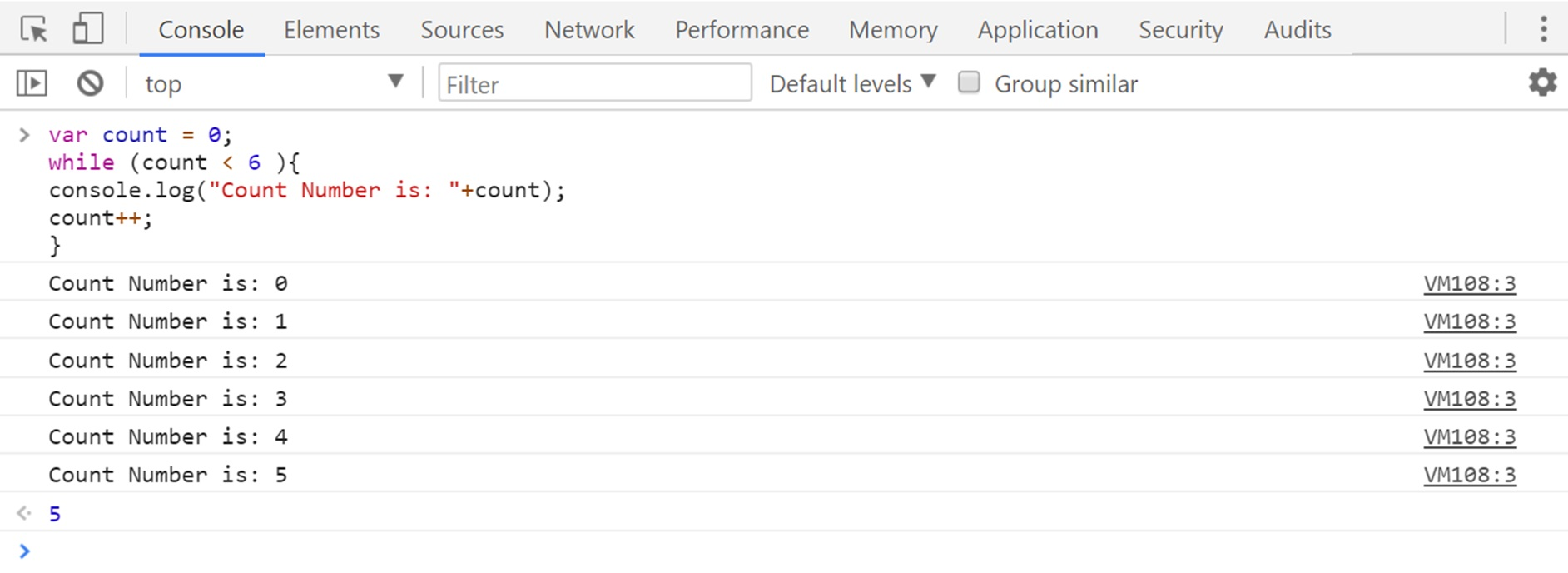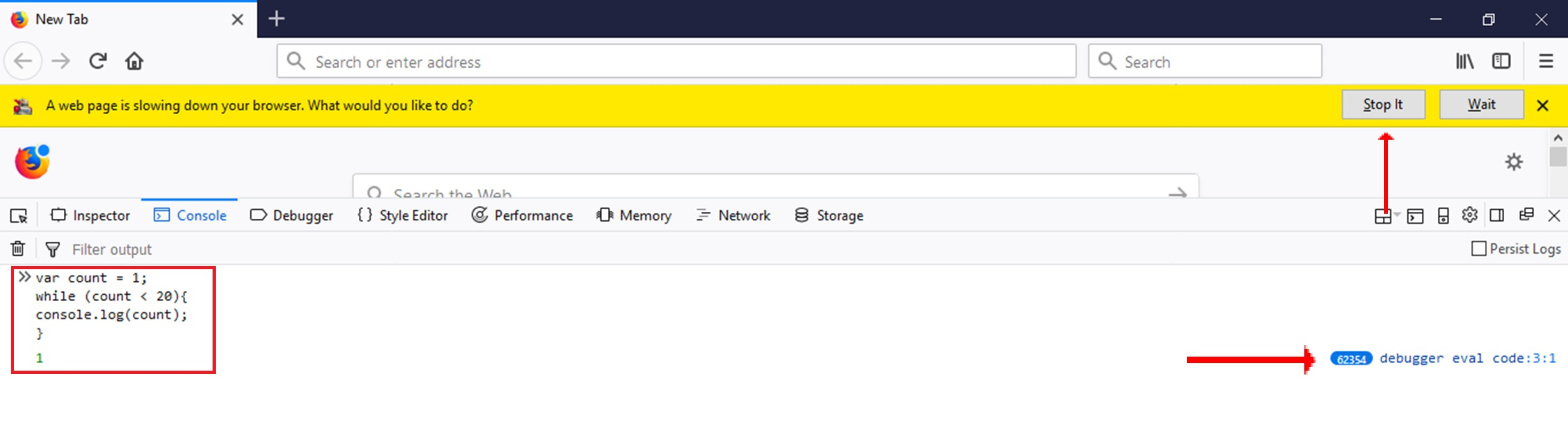# Lesson 11 Loops in JavaScript

In this lesson, we are going to focus on loops in JavaScript and going to discuss why we use loops and what their function, here is the objective for this lesson.

## Objectives

• Understand the purpose of loops
• Define “DRY” code
• Write simple while loops

## LOOPS

Loop in JavaScript programming is similar to other Programming Languages, so the loop is a block of code which continually executed until we found a required condition.
For example, if we want to print number from 0 to 5 on a different line, so I have to write 6 different console.log() in order to print 0 to 5 number.
Here is an example below:This is not the ideal way to write code.
So this is where loops come in.

So Loops are very portable if you want to execute the same code over and over again, each time with another value.
It can run a block of code a number of times, often used with arrays.

## DRY

So before we see the syntax of while loops in JavaScript, I want to introduce the concept called DRY code.
So DRY stands for Don’t Repeat yourself, it is basically a concept, which comes around almost every programming language, so the aim of this concept is to reduce repetition in code and make our code cleaner and also save a lot of our time.
So you can notice in above example that the code is very repetitive, the only difference that the number that we are printing out, so that code is not DRY code.
So we use Loops to DRY up our code.

## WHILE LOOPS

There are multiple types of loops.
Initially, we are discussing while loops.
While loops are much similar to IF statement, so it takes a condition like A less than 10 or answer not equal to and while that condition is true.
But WHILE loop continues to run the code as long as the condition is true.

## Syntax

``````while(Condition1) {
//This line of code will be executed there.
}
``````

## Example

Here is an example: we can print the numbers from 0 to 5 using a while loop.

var count = 0;

Then we have our while loop with a condition that says the count is less than 6.

while (count < 6 ){

So the first time this loop runs count is one, so 0 less than 6 which is true.
So this code is run and it will print “Count Number is: 0”

count++;

Then it will increment the value of count and add 1 to it, then it goes again and again.
You can play with the numbers here.

## JavaScript Code

``````var count = 0;
while (count < 6 ){
console.log("Count Number is: "+count);
count++;
}
``````

## Output

So here is the output of above JavaScript codeSo as you can probably see already a loop can help us save a lot of time, we don’t need to write statement multiple time if we are using Loops.
Yes, that’s a DRY code.

## Infinite Loops

Infinite loops occur when the condition that we provide is never false.

So it just keeps going and going forever.
This situation is really a big issue because it can crash the browser, it can take up all the memory in JavaScript.
Here is an example how one would happen.

## JavaScript Code

We have declared a count variable and assign value 1, and here is while condition which is ‘count less than 20’, which is obviously always going to less than 20, it is because we are not incrementing count value after console.log, so the count value will stay 1 and count value never going to be over 20.
So this will print 1 forever,

``````var count = 1;
while (count < 20){
console.log(count);
}
``````

I will not recommend you to do this, it is obviously going to crash your browser.

## Output

So in this output, you can notice when I run my infinite loop code since my variable value is 1 and we have not incremented count so the while statement will always be true and it will execute my code indefinitely.Here my JavaScript executed more than 60 thousand times and my browser asking me to stop it.
In this lesson, we have studied how we can use Loops in JavaScript and what’s function of Loops in Programming languages.
We have also discussed the concept of DRY code.